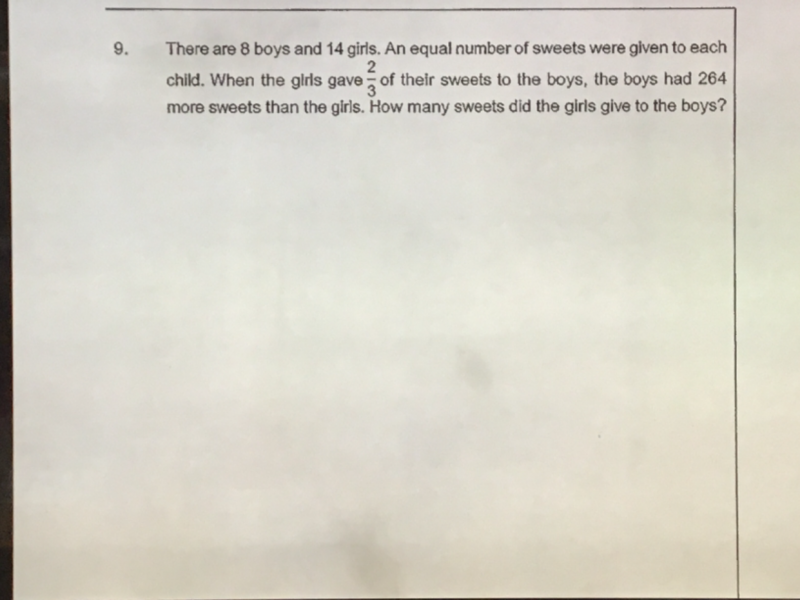# QuestionPls help to solve

I believe there is an error in this question.  The boys had 266 more sweets than the girls instead of 264…   264 will result in decimal instead of whole number.

u = number of sweets per child

Before:

G    [—————–14u——————-]

B    [——–8u————]

After:

G    [(1/3)(14u)]<————-266———————>

B    [——–8u————][—-(2/3)(14u)————]

(1/3)(14u) + 266 = 8u + (2/3)(14u)   <———— x 3 on both sides

14u + 798 = 24u + 28u

38u = 798

u = 21

Number of sweets girls have at first = 14u = 14 x 21 = 294

Number of sweets girls gave to the boys = (2/3) (294) = 196

PS: Aaron,  please check if the answer is correct and click on “Accept answer” if it is.

Let’s assume 308 is the correct answer.

u = number of sweets given to each child.

Boys = 8u,  Girls = 14u

(2/3)(14u) = 308

14u = 462 => u = 33

So Boys = 8 x 33 = 264 and Girls = 14 x 33 = 462

Girls leftover sweets = 462-308 = 154

Boys received 308 sweets from Girls =  264+308 = 572

So 572 – 154 = 418 <> 264  (as stated in the question, after receiving 2/3 sweets from girls, difference is 264)

Hence 308 answer key is incorrect.

0 Replies 0 Likes